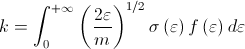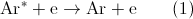# Simulation of Complex Rarefied Non-equilibrium Plasma Flows

### Introduction

The research is part of a DOE funded plasma science center focused on the control of plasma kinetics. We more specifically seek to investigate the possibility of controlling the electron energy distribution function (EEDF) in a flow of cold rarefied Argon plasma. The overarching goal of the project is to improve the ability to predict the EEDF in cold plasma flows where the neutrals are in the rarefied regime (i.e. governed by kinetic equations). This will be accomplished by both measuring and simulating the EEDF for the test case detailed below with the ultimate goal of being able to reproduce numerically experimental measurements.

Why try to shape the EEDF ?

Many industrial processes involving low temperature plasmas (such as etching or deposition) are based on the interaction of excited atoms or molecules with solid surfaces. Excited states are created as the results of the collisions of atoms or molecules with electrons which motion is itself driven by an externally imposed electric field. The rate of the reactions of practical interest k is often limited by the cross section of the activation reaction σ which can be written:When the cross section σ  is fixed, the value of the rate k can be maximized by changing f(ε) so as to increase the value of the integral. The shape of the EEDF can be altered by modifying the relative population of electron energy levels. In the present case, we seek to increase the proportion of higher energetic electrons.

How to shape the EEDF ?

This is accomplished by injecting Argon metastables (denoted Ar*), which are excited Argon atoms with greater total energy due to their electronic configuration. These in turn take part in the following deexcitation reaction:This reaction transfers energy from Ar* to the electron thereby increasing the proportion of more energetic electrons. This results in an increase of the tail of the EEDF.

### Test Case

The test case being simulated  is shown below. It corresponds to an actual experiment that is being conducted at PEPL. It consists of two counter-flowing jets which ultimate goal is to put electrons in contact with Ar so that reaction (1) can occur.Figure 1: Test Case Overview.

### Simulation Method & Results

Argon atoms, due to the very low pressure of the vacuum chamber are in the rarefied regime characterized by large Knudsen number. Their dynamics are thus governed by the Boltzmann equation which is simulated via the Direct Simulation Monte Carlo (DSMC) method within the framework of MONACO. Electrons are simulated with a fluid model which will later be extended to account for non-Maxwellian EEDF.

The first plot below shows the number density of Ar and Ar* emanating from the two counterflowing jets. The second plot shows the plasma potential as obtained by the detailed fluid model.Figure 2: Number density of Ar and Ar* [m-3 ].Figure 3: Plasma potential ϕ[V] between the two jets.

### Acknowledgement

Financial support from the Department of Energy's Office of Science (Grant SC0001939) is gratefully acknowledged.